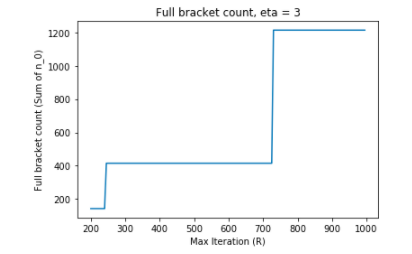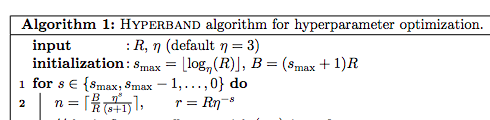# Tune Trial Schedulers (tune.schedulers)#

In Tune, some hyperparameter optimization algorithms are written as “scheduling algorithms”. These Trial Schedulers can early terminate bad trials, pause trials, clone trials, and alter hyperparameters of a running trial.

All Trial Schedulers take in a `metric`, which is a value returned in the result dict of your Trainable and is maximized or minimized according to `mode`.

```from ray import train, tune
from tune.schedulers import ASHAScheduler

def train_fn(config):
# This objective function is just for demonstration purposes
train.report({"loss": config["param"]})

tuner = tune.Tuner(
train_fn,
tune_config=tune.TuneConfig(
scheduler=ASHAScheduler(),
metric="loss",
mode="min",
num_samples=10,
),
param_space={"param": tune.uniform(0, 1)},
)
results = tuner.fit()
```

## ASHA (tune.schedulers.ASHAScheduler)#

The ASHA scheduler can be used by setting the `scheduler` parameter of `tune.TuneConfig`, which is taken in by `Tuner`, e.g.

```from ray import tune
from tune.schedulers import ASHAScheduler

asha_scheduler = ASHAScheduler(
time_attr='training_iteration',
metric='loss',
mode='min',
max_t=100,
grace_period=10,
reduction_factor=3,
brackets=1,
)
tuner = tune.Tuner(
train_fn,
tune_config=tune.TuneConfig(scheduler=asha_scheduler),
)
results = tuner.fit()
```

Compared to the original version of HyperBand, this implementation provides better parallelism and avoids straggler issues during eliminations. We recommend using this over the standard HyperBand scheduler. An example of this can be found here: Asynchronous HyperBand Example.

Even though the original paper mentions a bracket count of 3, discussions with the authors concluded that the value should be left to 1 bracket. This is the default used if no value is provided for the `brackets` argument.

 `AsyncHyperBandScheduler`([time_attr, metric, ...]) Implements the Async Successive Halving. `ASHAScheduler`

## HyperBand (tune.schedulers.HyperBandScheduler)#

Tune implements the standard version of HyperBand. We recommend using the ASHA Scheduler over the standard HyperBand scheduler.

 `HyperBandScheduler`([time_attr, metric, ...]) Implements the HyperBand early stopping algorithm.

### HyperBand Implementation Details#

Implementation details may deviate slightly from theory but are focused on increasing usability. Note: `R`, `s_max`, and `eta` are parameters of HyperBand given by the paper. See this post for context.

1. Both `s_max` (representing the `number of brackets - 1`) and `eta`, representing the downsampling rate, are fixed.

In many practical settings, `R`, which represents some resource unit and often the number of training iterations, can be set reasonably large, like `R >= 200`. For simplicity, assume `eta = 3`. Varying `R` between `R = 200` and `R = 1000` creates a huge range of the number of trials needed to fill up all brackets.On the other hand, holding `R` constant at `R = 300` and varying `eta` also leads to HyperBand configurations that are not very intuitive:The implementation takes the same configuration as the example given in the paper and exposes `max_t`, which is not a parameter in the paper.

1. The example in the post to calculate `n_0`

is actually a little different than the algorithm given in the paper. In this implementation, we implement `n_0` according to the paper (which is `n` in the below example):1. There are also implementation specific details like how trials are placed into brackets which are not covered in the paper.

This implementation places trials within brackets according to smaller bracket first - meaning that with low number of trials, there will be less early stopping.

## Median Stopping Rule (tune.schedulers.MedianStoppingRule)#

The Median Stopping Rule implements the simple strategy of stopping a trial if its performance falls below the median of other trials at similar points in time.

 `MedianStoppingRule`([time_attr, metric, ...]) Implements the median stopping rule as described in the Vizier paper:

## Population Based Training (tune.schedulers.PopulationBasedTraining)#

Tune includes a distributed implementation of Population Based Training (PBT). This can be enabled by setting the `scheduler` parameter of `tune.TuneConfig`, which is taken in by `Tuner`, e.g.

```from ray import tune
from ray.tune.schedulers import PopulationBasedTraining

pbt_scheduler = PopulationBasedTraining(
time_attr='training_iteration',
metric='loss',
mode='min',
perturbation_interval=1,
hyperparam_mutations={
"lr": [1e-3, 5e-4, 1e-4, 5e-5, 1e-5],
"alpha": tune.uniform(0.0, 1.0),
}
)
tuner = tune.Tuner(
train_fn,
tune_config=tune.TuneConfig(
num_samples=4,
scheduler=pbt_scheduler,
),
)
tuner.fit()
```

When the PBT scheduler is enabled, each trial variant is treated as a member of the population. Periodically, top-performing trials are checkpointed (this requires your Trainable to support save and restore). Low-performing trials clone the hyperparameter configurations of top performers and perturb them slightly in the hopes of discovering even better hyperparameter settings. Low-performing trials also resume from the checkpoints of the top performers, allowing the trials to explore the new hyperparameter configuration starting from a partially trained model (e.g. by copying model weights from one of the top-performing trials).

Take a look at Visualizing Population Based Training (PBT) Hyperparameter Optimization to get an idea of how PBT operates. A Guide to Population Based Training with Tune gives more examples of PBT usage.

 `PopulationBasedTraining`([time_attr, metric, ...]) Implements the Population Based Training (PBT) algorithm.

## Population Based Training Replay (tune.schedulers.PopulationBasedTrainingReplay)#

Tune includes a utility to replay hyperparameter schedules of Population Based Training runs. You just specify an existing experiment directory and the ID of the trial you would like to replay. The scheduler accepts only one trial, and it will update its config according to the obtained schedule.

```from ray import tune
from ray.tune.schedulers import PopulationBasedTrainingReplay

replay = PopulationBasedTrainingReplay(
experiment_dir="~/ray_results/pbt_experiment/",
trial_id="XXXXX_00001"
)
tuner = tune.Tuner(
train_fn,
tune_config=tune.TuneConfig(scheduler=replay)
)
results = tuner.fit()
```

See here for an example on how to use the replay utility in practice.

 `PopulationBasedTrainingReplay`(policy_file) Replays a Population Based Training run.

## Population Based Bandits (PB2) (tune.schedulers.pb2.PB2)#

Tune includes a distributed implementation of Population Based Bandits (PB2). This algorithm builds upon PBT, with the main difference being that instead of using random perturbations, PB2 selects new hyperparameter configurations using a Gaussian Process model.

The Tune implementation of PB2 requires GPy and sklearn to be installed:

```pip install GPy sklearn
```

PB2 can be enabled by setting the `scheduler` parameter of `tune.TuneConfig` which is taken in by `Tuner`, e.g.:

```from ray.tune.schedulers.pb2 import PB2

pb2_scheduler = PB2(
time_attr='time_total_s',
metric='mean_accuracy',
mode='max',
perturbation_interval=600.0,
hyperparam_bounds={
"lr": [1e-3, 1e-5],
"alpha": [0.0, 1.0],
...
}
)
tuner = tune.Tuner( ... , tune_config=tune.TuneConfig(scheduler=pb2_scheduler))
results = tuner.fit()
```

When the PB2 scheduler is enabled, each trial variant is treated as a member of the population. Periodically, top-performing trials are checkpointed (this requires your Trainable to support save and restore). Low-performing trials clone the checkpoints of top performers and perturb the configurations in the hope of discovering an even better variation.

The primary motivation for PB2 is the ability to find promising hyperparamters with only a small population size. With that in mind, you can run this PB2 PPO example to compare PB2 vs. PBT, with a population size of `4` (as in the paper). The example uses the `BipedalWalker` environment so does not require any additional licenses.

 `pb2.PB2`([time_attr, metric, mode, ...]) Implements the Population Based Bandit (PB2) algorithm.

## BOHB (tune.schedulers.HyperBandForBOHB)#

This class is a variant of HyperBand that enables the BOHB Algorithm. This implementation is true to the original HyperBand implementation and does not implement pipelining nor straggler mitigation.

This is to be used in conjunction with the Tune BOHB search algorithm. See TuneBOHB for package requirements, examples, and details.

An example of this in use can be found here: BOHB Example.

 `HyperBandForBOHB`([time_attr, metric, mode, ...]) Extends HyperBand early stopping algorithm for BOHB.

## ResourceChangingScheduler#

This class is a utility scheduler, allowing for trial resource requirements to be changed during tuning. It wraps around another scheduler and uses its decisions.

• If you are using the Trainable (class) API for tuning, your Trainable must implement `Trainable.update_resources`,

which will let your model know about the new resources assigned. You can also obtain the current trial resources by calling `Trainable.trial_resources`.

• If you are using the functional API for tuning, get the current trial resources obtained by calling

`tune.get_trial_resources()` inside the training function. The function should be able to load and save checkpoints (the latter preferably every iteration).

An example of this in use can be found here: XGBoost Dynamic Resources Example.

 `ResourceChangingScheduler`(base_scheduler, ...) A utility scheduler to dynamically change resources of live trials. This class creates a basic uniform resource allocation function. This class creates a "TopJob" resource allocation function.

## FIFOScheduler (Default Scheduler)#

 Simple scheduler that just runs trials in submission order.

## TrialScheduler Interface#

 Interface for implementing a Trial Scheduler class.
 Called to choose a new trial to run. Called on each intermediate result returned by a trial. Notification for the completion of trial.

## Shim Instantiation (tune.create_scheduler)#

There is also a shim function that constructs the scheduler based on the provided string. This can be useful if the scheduler you want to use changes often (e.g., specifying the scheduler via a CLI option or config file).

 `create_scheduler`(scheduler, **kwargs) Instantiate a scheduler based on the given string.